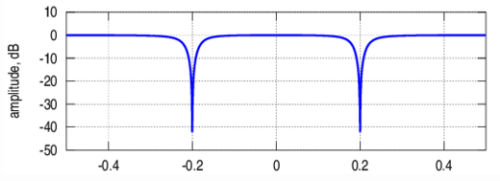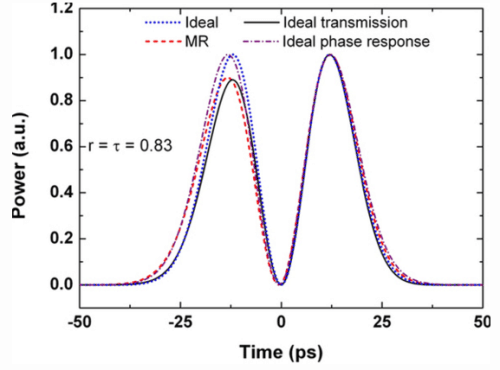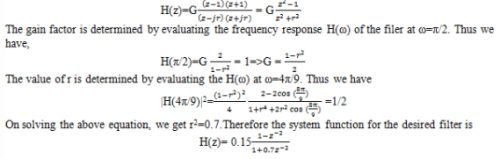Test: Frequency Selective Filters

# Test: Frequency Selective Filters

Test Description

## 10 Questions MCQ Test Digital Signal Processing | Test: Frequency Selective Filters

Test: Frequency Selective Filters for Electrical Engineering (EE) 2023 is part of Digital Signal Processing preparation. The Test: Frequency Selective Filters questions and answers have been prepared according to the Electrical Engineering (EE) exam syllabus.The Test: Frequency Selective Filters MCQs are made for Electrical Engineering (EE) 2023 Exam. Find important definitions, questions, notes, meanings, examples, exercises, MCQs and online tests for Test: Frequency Selective Filters below.
Solutions of Test: Frequency Selective Filters questions in English are available as part of our Digital Signal Processing for Electrical Engineering (EE) & Test: Frequency Selective Filters solutions in Hindi for Digital Signal Processing course. Download more important topics, notes, lectures and mock test series for Electrical Engineering (EE) Exam by signing up for free. Attempt Test: Frequency Selective Filters | 10 questions in 10 minutes | Mock test for Electrical Engineering (EE) preparation | Free important questions MCQ to study Digital Signal Processing for Electrical Engineering (EE) Exam | Download free PDF with solutions
 1 Crore+ students have signed up on EduRev. Have you?
Test: Frequency Selective Filters - Question 1

### If the system has a impulse response as h(n)=Asin(n+1)ω0u(n), then the system is known as Digital frequency synthesizer.

Detailed Solution for Test: Frequency Selective Filters - Question 1

Explanation: The given impulse response is h(n)=Asin(n+1)ω0u(n).
According to the above equation, the second order system with complex conjugate poles on the unit circle is a sinusoid and the system is called a digital sinusoidal oscillator or a Digital frequency synthesizer.

Test: Frequency Selective Filters - Question 2

### The filter with the system function H(z)=z -k is a:

Detailed Solution for Test: Frequency Selective Filters - Question 2

Explanation: The system with the system function given as H(z)=z -k is a pure delay system . It has a constant gain for all frequencies and hence called as All pass filter.

Test: Frequency Selective Filters - Question 3

### A comb filter is a special case of notch filter in which the nulls occur periodically across the frequency band.

Detailed Solution for Test: Frequency Selective Filters - Question 3

Explanation: A comb filter can be viewed as a notch filter in which the nulls occur periodically across the frequency band, hence the analogy to an ordinary comb that has periodically spaced teeth.

Test: Frequency Selective Filters - Question 4

Which of the following filters have a frequency response as shown below?Detailed Solution for Test: Frequency Selective Filters - Question 4

Explanation: The given figure represents the frequency response characteristic of a notch filter with nulls at frequencies at ω0 and ω1.

Test: Frequency Selective Filters - Question 5

A digital resonator is a special two pole band pass filter with the pair of complex conjugate poles located near the unit circle.

Detailed Solution for Test: Frequency Selective Filters - Question 5

Explanation: The magnitude response of a band pass filter with two complex poles located near the unit circle is as shown below.The filter gas a large magnitude response at the poles and hence it is called as digital resonator.

Test: Frequency Selective Filters - Question 6

If the low pass filter described by the difference equation y(n)=0.9y(n-1)+0.1x(n) is converted into a high pass filter, then what is the frequency response of the high pass filter?

Detailed Solution for Test: Frequency Selective Filters - Question 6

Explanation: The difference equation for the high pass filter is
y(n)=-0.9y(n-1)+0.1x(n)
and its frequency response is given as
H(ω)= 0.1/(1+0.9e-jω).

Test: Frequency Selective Filters - Question 7

If hlp(n) denotes the impulse response of a low pass filter with frequency response Hlp(ω), then what is the frequency response of the high pass filter in terms of Hlp(ω)?

Detailed Solution for Test: Frequency Selective Filters - Question 7

Explanation: The impulse response of a high pass filter is simply obtained from the impulse response of the low pass filter by changing the signs of the odd numbered samples in hlp(n). Thus
hhp(n)=(-1)n hlp(n)=(e)n hlp(n)
Thus the frequency response of the high pass filter is obtained as Hlp(ω-π).

Test: Frequency Selective Filters - Question 8

What is the system function for a two pole band pass filter that has the centre of its pass band at ω=π/2, zero its frequency response characteristic at ω=0 and at ω=π, and its magnitude response is 1/√2 at ω=4π/9?

Detailed Solution for Test: Frequency Selective Filters - Question 8

Explanation: Clearly, the filter must have poles at P1,2=re±jπ/2 and zeros at z=1 and z=-1. Consequently the system function isTest: Frequency Selective Filters - Question 9

If the phase ϴ(ω) of the system is linear, then the group delay of the system:

Detailed Solution for Test: Frequency Selective Filters - Question 9

Explanation: We know that the group delay of the system with phase ϴ(ω) is defined as
Tg(ω)=(dϴ(ω))/dω
Given the phase is linear=> the group delay of the system is constant.

Test: Frequency Selective Filters - Question 10

An ideal filter should have zero gain in their stop band.

Detailed Solution for Test: Frequency Selective Filters - Question 10

Explanation: For an ideal filter, in the magnitude response plot at the stop band it should have a sudden fall which means an ideal filter should have a zero gain at stop band.

## Digital Signal Processing

3 videos|50 docs|54 tests
 Use Code STAYHOME200 and get INR 200 additional OFF Use Coupon Code
Information about Test: Frequency Selective Filters Page
In this test you can find the Exam questions for Test: Frequency Selective Filters solved & explained in the simplest way possible. Besides giving Questions and answers for Test: Frequency Selective Filters, EduRev gives you an ample number of Online tests for practice

## Digital Signal Processing

3 videos|50 docs|54 tests